The Frailty Model

You can use the frailty model to model correlations between failures of the same cluster by using a random component for the hazard function. The hazard rate for theth individual in theth cluster iswhere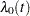is an arbitrary baseline hazard rate,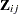is the vector of (fixed-effect) covariates,is the vector of regression coefficients, and
is the random effect for cluster. The random componentsare assumed to be independent and identically distributed as a normal random variable with mean 0 and an unknown variance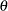.

In terms of the frailties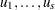, given by, the frailty model can be written as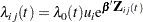Each frailty has a lognormal distribution with median 1. This gives the interpretation that individuals in clusterwithtend to fail at a faster (slower) rate than that under an independence model.

The RANDOM statement in PROC PHREG enables you to fit a shared fraity model. However, the ASSESS, BASELINE, and OUTPUT statements, if specified, are ignored. Also ignored are the COVS options in the PROC PHREG statement and the following options in the MODEL statement: BEST=, DETAILS, HIERARCHY=, INCLUDE=, NOFIT, PLCONV=, SELECTION=, SEQUENTIAL, SLENTRY=, SLSTAY=, TYPE1, and TYPE3(ALL, LR, SCORE). Profile likelihood confidence intervals for the hazard ratios are not available for the frailty model analysis.

### The Penalized Partial Likelihood Approach for Fitting Frailty Models

Letbe the vector of random components for theclusters. With eachhaving a zero-mean normal distribution and a common variance, the joint log likelihood is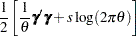Define the penalized partial log likelihood as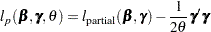whereis the log of any of the partial likelihood in the sections Partial Likelihood Function for the Cox Model and The Multiplicative Hazards Model.

For a given, let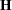be the negative Hessian of the penalized partial log likelihood; that is,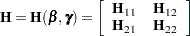where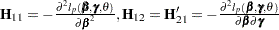, and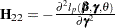.

The marginal log likelihood of this shared frailty model isUsing a Laplace approximation to the integral as in Breslow and Clayton (1993), an approximate marginal log likelihood (Ripatti and Palmgren; 2000; Therneau and Grambsch; 2000) is given byThe maximization of this approximate likelihood is a doubly iterative process that alternates between the following two steps:

• For a provisional value of, PROC PHREG computes the best linear unbiased predictors (BLUP) ofandby maximizing the penalized partial log likelihood. This contitutes the inner loop.

• Forandfixed at the BLUP values, PROC PHREG estimatesby maximizing the approximate marginal likelihood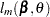. This constitutes the outer loop.

The outer loop is iterated until the difference between two successive estimates ofis small.

The ML estimate ofisThe variance for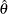is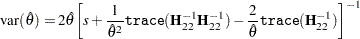The REML estimation ofis obtained by replacing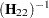by.

The inverse of the finalmatrix is used as the variance estimate of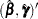.

The final BLUP estimates of the random componentscan be displayed using the SOLUTION option in the RANDOM statement. Also displayed are estimates of the lognormal frailties, which are the exponentiated estimates of the BLUP estimates.

### Wald-Type Tests for Penalized Models

Letbe the negative Hessian of the partial log likelihood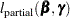:where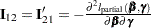, and. Write. The Wald-type chi-square statistic for testing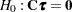is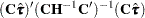Let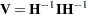. Gray (1992) recommends the following generalized degrees of freedom for the Wald test: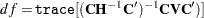See Therneau and Grambsch (2000, Section 5.8) for a discussion of this Wald-type test.

PROC PHREG uses the label "Adjusted DF" to represent this generalized degrees of freedom in the output.